# What is Linear Regression

Linear: The relationship between two variables is a linear function (such as x and y, all input data and all output results) - the graph is a straight line, called linear.
Regression: Predicted value. output continuous value

Predict the outcome. Get unknown results from known data. For example: prediction of house price, judgment of credit evaluation, movie box office prediction, etc.

# specific content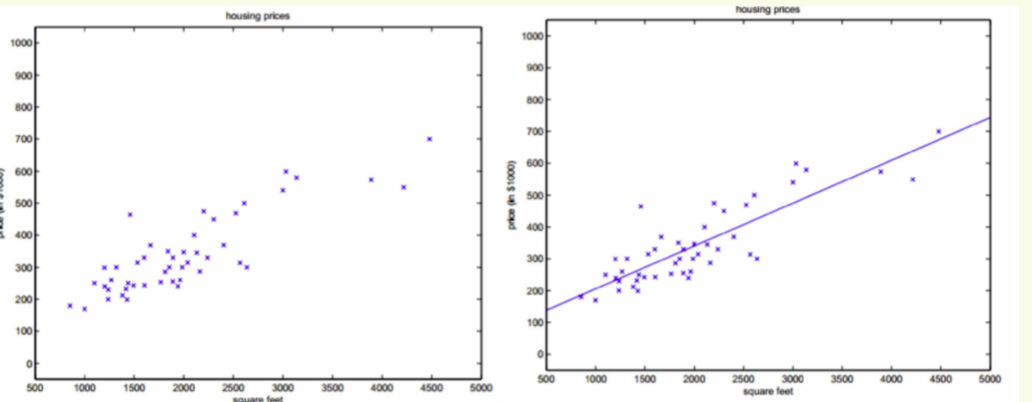By simulating such a straight line, then when new input data x comes in, through this straight line/function (model), we can get the output y

The general model of linear regression: (function)
is like y=kx, x is the input data, y is the output result, and k is the parameter we require.

Here x0,x1,x2. . . . . It is the different characteristics of the data.
All you need is the value of theta. thet is the parameter we require, and it is also a parameter that needs to be continuously optimized in the future.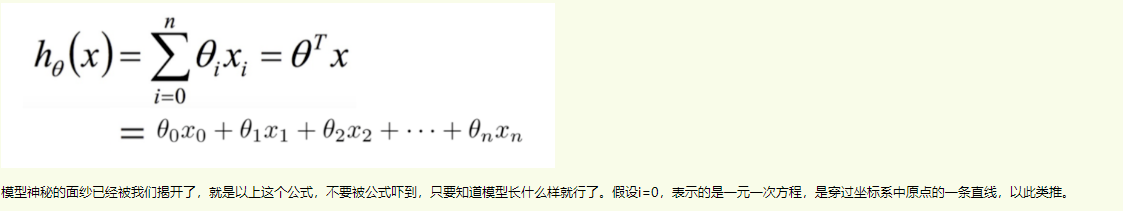## Parameter calculation

Bring in a set of x, y to get the parameters of the function. After the prediction function is obtained, the parameters of the function need to be optimized. Introduce a loss function.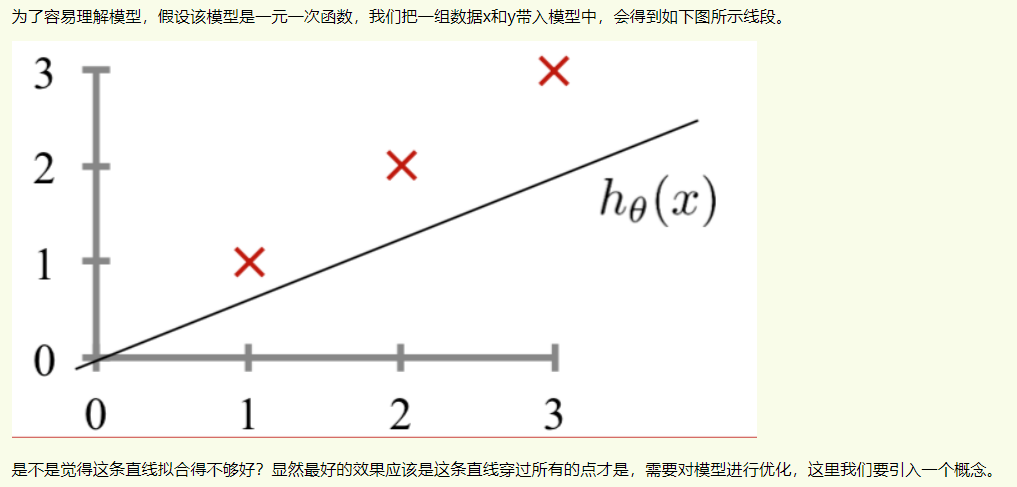Loss function: It is used to measure the inconsistency between the predicted value f(x) of your model and the true value YY. The smaller the loss function, the better the effect of the model.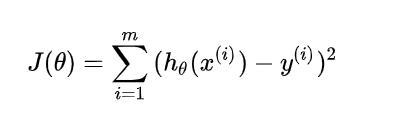Average of sums of squares (predicted value - true value)

How to make the loss function smaller - using gradient descent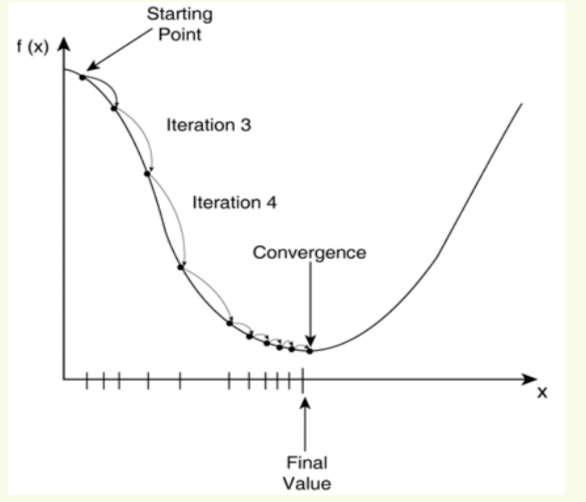The loss function is relatively large at the beginning, but with the continuous change of the straight line (the model is continuously trained), the loss function will become smaller and smaller, so as to reach the minimum point, which is the final model we want to get.

This method is collectively referred to as the gradient descent method: with the continuous training of the model, the gradient of the loss function becomes more and more flat, until the minimum point, the distance between the point and the line is the smallest, so the line will pass through all the points. , which is the model (function) we ask for.

By analogy, the high-dimensional linear regression model is the same. The gradient descent method is used to optimize the model and find the extreme point (this point is the parameter we require), which is the process of model training.

# What is logistic regression? (what is the problem)

Although Logistic regression has "regression" in its name, it is actually a classification method, mainly used for two-classification problems (that is, there are only two outputs, representing two categories respectively)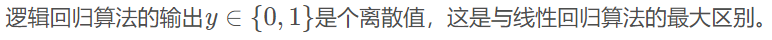Logistic regression is to set a function outside the output y of linear regression, so that the final output result is a classification of 0 or 1. Because the linear regression output value y is a continuous value.

We do another functional transformation on the output y of this linear regression to become g(y). If we let the value of g(y) be class A in one real interval, class B in another real interval, and so on, we get a classification model.

# Specific content (how to solve it?)

## Build a prediction function (linear regression sets another function)

The predict function has its value output between [ 0 , 1 ]. That is, the output of the prediction function is a continuous value! ! For example, 0.8. Then select a threshold, such as 0.5, if the calculated predicted value is greater than 0.5, and 0.8 is greater than 0.5, the predicted value is considered to be 1, otherwise, the predicted value is 0.

### Sigmoid function (Logistic function)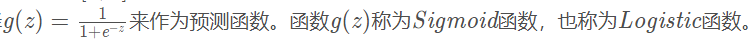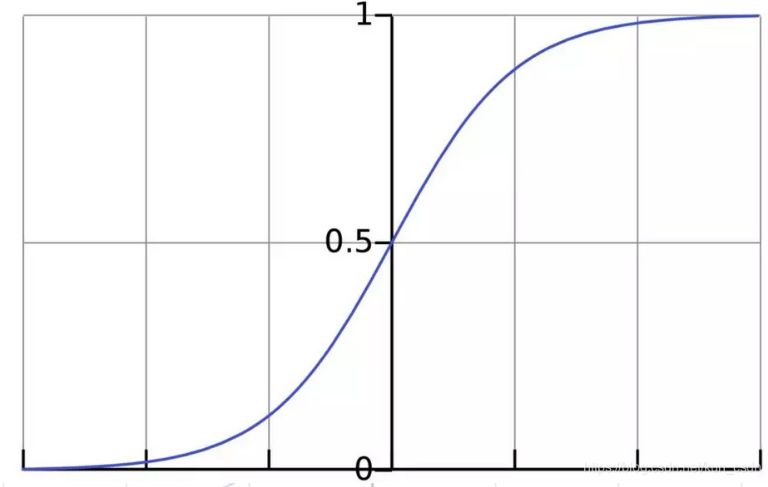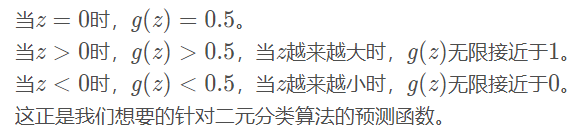## loss function

However, since logistic regression is not continuous, the experience of defining the loss function of natural linear regression cannot be used. However, we can use the maximum likelihood method to derive our loss function.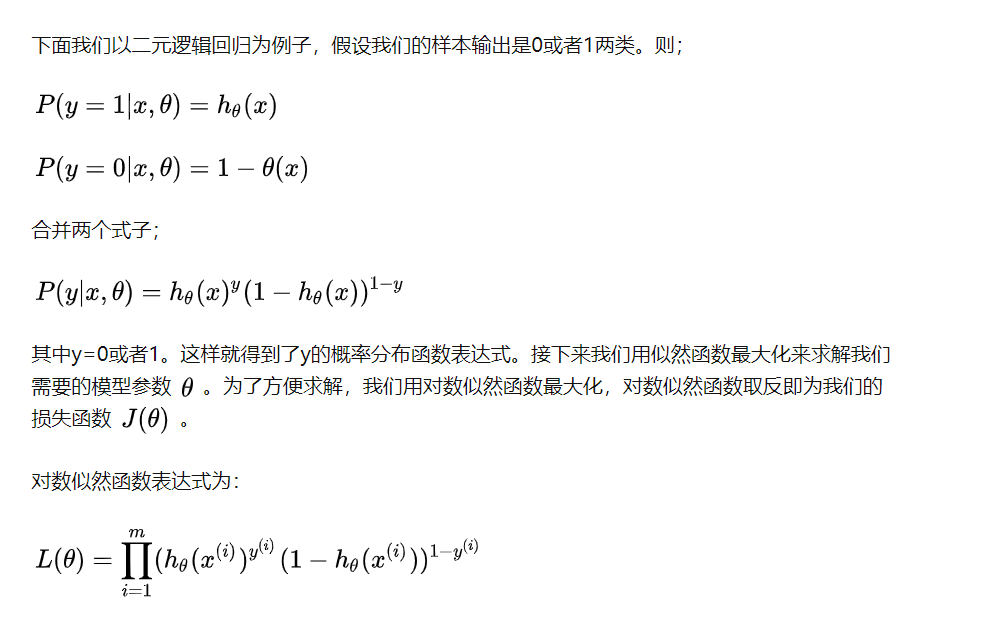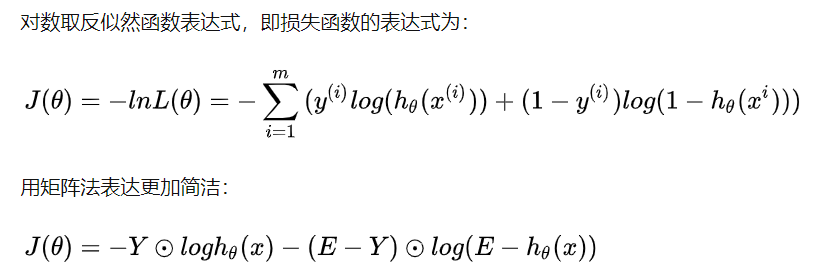## Loss function optimization method for logistic regression

Through continuous optimization, find the best parameter theta.
For the minimization of the loss function of binary logistic regression, there are many methods, the most common ones are gradient descent method, coordinate axis descent method, Newton method, etc. Here the formula for each iteration of θ in gradient descent is derived. Due to the cumbersome derivation and comparison of the algebraic method, I am accustomed to using the matrix method to optimize the loss function. Here is the process of deriving the gradient of binary logistic regression by the matrix method.

## multivariate classification

The logistic regression model can solve the binary classification problem, that is, y = { 0 , 1 } . Can it be used to solve the multivariate classification problem? The answer is yes. For multivariate classification problems, y = { 0 , 1 , 2 , . . . , n } , with a total of n + 1 classes. The solution is to first convert the problem into a binary classification problem, that is, y = 0 {y=0}y=0 is a category, y = { 1 , 2 , . . . , n } as another category, and then calculate The probabilities of these two classes; then, taking y = 1 as one class and y = { 0 , 2 , . . . , n } as the other class, calculate the probabilities of these two classes. Generalizing from this, a total of n+1 prediction functions (classifiers) are required. The category with the highest predicted probability is the category to which the sample belongs.

For there are three categories. That is, for a sample, calculate the probability of belonging to the 0 category and the probability of not belonging to 0. Calculate the probability of belonging to category 1 and the probability of not belonging to 1. Calculate the probability of belonging to category 2 and the probability of not belonging to 2. The last one with the highest probability is the category to which the sample belongs.

# What is the use of logistic regression? (what result)

Looking for risk factors: looking for risk factors for a disease, etc.;
Prediction: According to the model, predict the probability of a certain disease or a certain situation under different independent variables;
Discrimination: It is actually somewhat similar to prediction, but also based on Model, to judge the probability of someone belonging to a certain disease or a certain situation, that is, to see how likely this person is to belong to a certain disease.

1) Fast speed, suitable for binary classification problems
2) Simple and easy to understand, directly see the weight of each feature
3) Can easily update the model to absorb new data

## shortcoming

The ability to adapt to data and scenarios is limited, not as adaptable as the decision tree algorithm

# Model overfitting

## Reasons for overfitting

1. Too many features and not enough data.
For regression algorithms, more features means more parameters (theta parameters), and the model is more complex .
In contrast, if the amount of data is insufficient, it will lead to overfitting, that is, the complexity of the model and the data The amount does not match.

2. The data characteristics and distribution of the training set and test set are not similar enough. The fundamental reason for this is that the training set is too small. In the overall sample, the training set and test set only account for a small part, which makes it difficult to guarantee the training set and test set. Similar to the overall data distribution, it is more difficult to ensure that the training set is similar to the test set distribution, which will cause the model to fully learn the characteristics of the training set and overfit, so the generalization ability of the model is definitely not enough.

3. Overtraining. When the model is overtrained on the training set, the model fully learns all the data features on this data set, which is overly sensitive to noise and outliers outliers, resulting in overfitting.

## Solution

Overfitting is because the model is too complex.

The solution is to reduce the number of input features, or get more training samples.

Regularization is also a method used to solve the problem of model overfitting.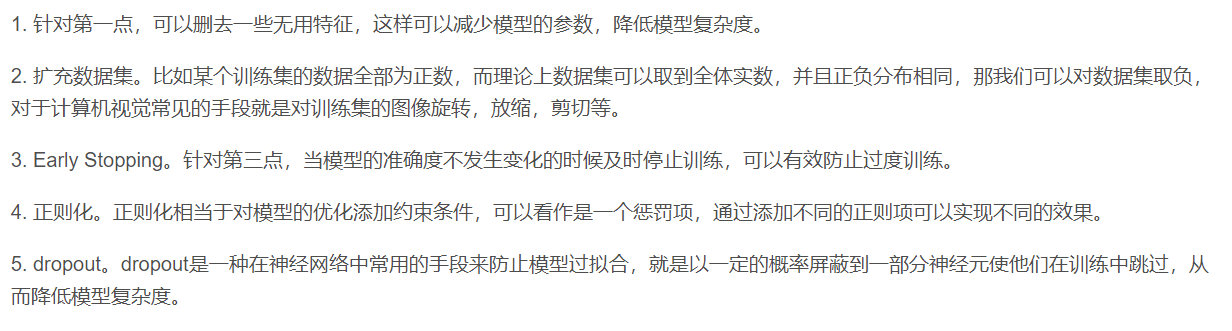## Regularization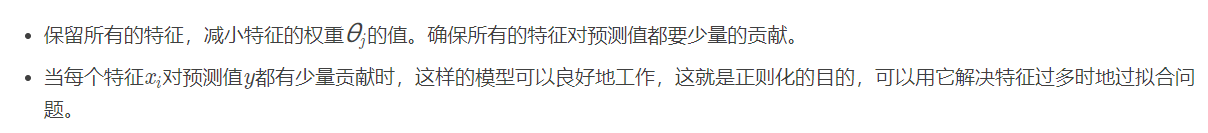The purpose of regularization is to make every feature in the data contribute a small amount to our predicted value, not be very biased towards certain weights, each feature has a little, such a model can work well.

Regularization can be used to solve the problem of overfitting when there are too many features .
Regularization (effective when there are many features) - keep all features, but reduce the size of theta (L2 regularization)
- remove some features (L1 regularization)

Specific operation: Add a regular term function after the loss function.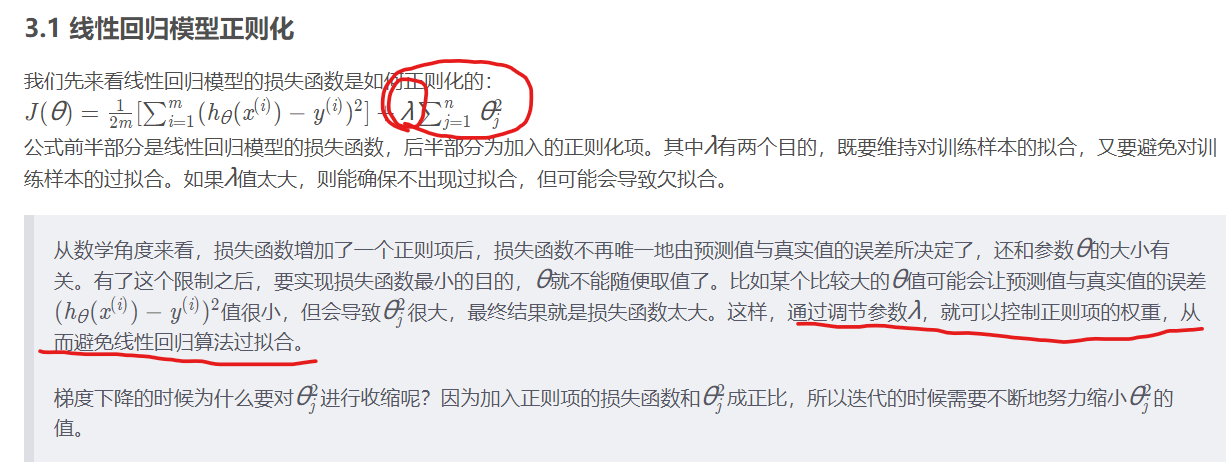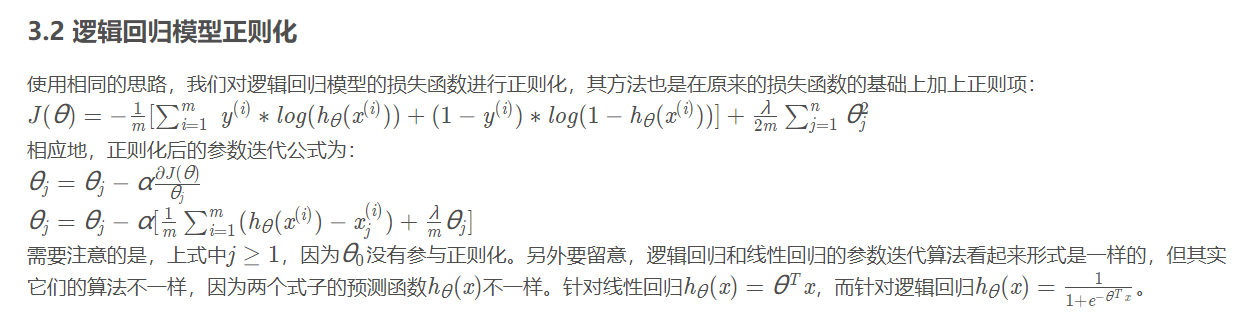### L1 regular term

Equivalent to feature filtering

L1 regularization makes the weights lean toward 0, making the weights in the network as 0 as possible , which is equivalent to reducing the complexity of the network and preventing overfitting.

This is why L1 regularization produces sparser solutions. Here sparsity means that some parameters in the optimal value are 0. The sparse nature of L1 regularization has been widely used in feature selection mechanisms to select meaningful features from a subset of available features.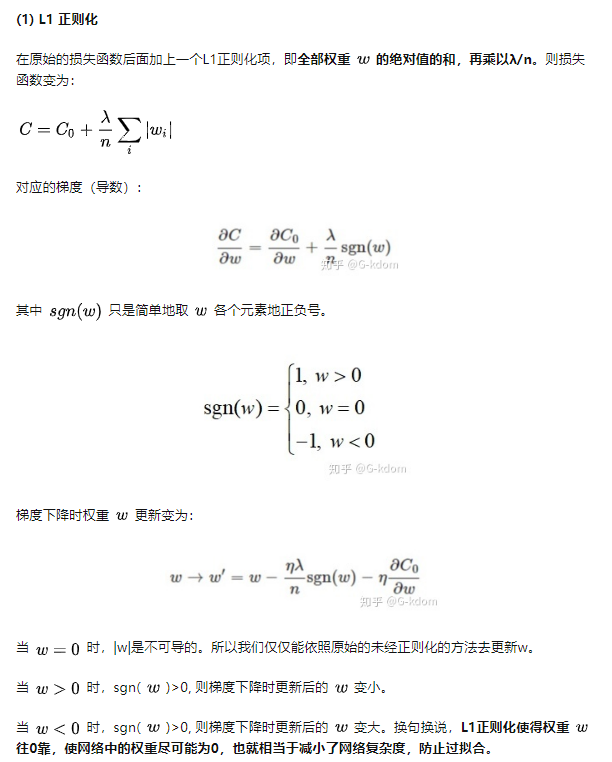### L2 regular term

Equivalent to feature sparse (all features are present, but each feature has fewer points, uniform points, feature decay )
in the process of gradient descent, the weight will gradually decrease, tending to 0 but not equal to 0. This is where the weight decay comes from.

L2 regularization has the effect of making the weight parameter smaller. Why can it prevent overfitting? Because the smaller weight parameter means that the complexity of the model is lower, the fitting to the training data is just right, and the training data will not be overfitted, thereby improving the generalization ability of the model.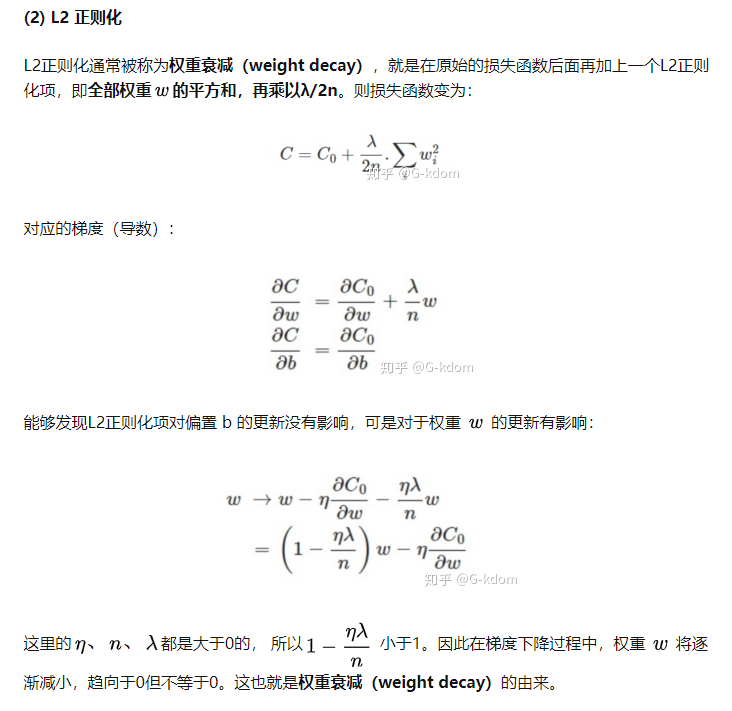# Model evaluation

True Yangs: True Yangs—predicted positive samples, and actually positive samples.
False Positives: False Positives - Predicted as positive, but actually negative.

True Yin: True Yin - Predicted as a negative sample, and actually is a negative sample.
False Negatives: False Negatives - Predicted negative samples, but actually positive samples.

Precision: The precision is for our predictions . How many of the predicted positive samples are really positive samples (of all predicted good melons, what proportion are really good melons? Of the predicted patients, how many are real patients). Precise means to see whether the results of our predictions are accurate or not.

Recall: The recall is for our original sample . Of all the really good melons, what percentage of good melons are predicted correctly? (out of all patients, what percentage of patients were predicted by us)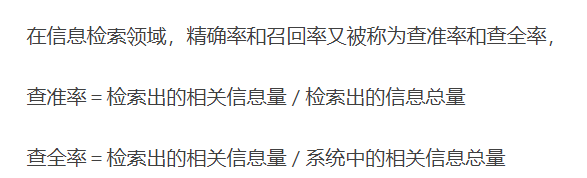## ROC curve+PR curve

ROC curves are meaningless to use in multiclass classification. Only when Positive and Negative are equally important in binary classification, it is suitable to use ROC curve evaluation.

The ROC curve is very commonly used as an evaluation metric in binary classification problems . But on very skewed datasets, the Precision-Recall (PR) Curve can give us a comprehensive understanding of the performance of the model.

ROC focuses on two indicators,
with TPR (true positive rate) as the y-axis and FPR (false positive rate) as the x-axis, we directly get the RoC curve.
TPR represents the probability that a positive example can be classified as a pair, and FPR represents the probability that a negative example can be mistakenly classified as a positive example. In the ROC space, the abscissa of each point is FPR and the ordinate is TPR, which also depicts the trade-off between TP (true rate) and FP (false positive rate) of the classifier.

It can be understood from the definitions of FPR and TPR that the higher the TPR and the smaller the FPR, the more efficient our models and algorithms will be.

The closer the line is to the upper left corner, it means that more positive examples are given priority over negative examples, and the overall performance of the model is better.

That is, the closer the drawn RoC curve is to the upper left, the better . As shown in the left image below. Geometrically speaking, the larger the area under the RoC curve, the better the model . So sometimes we use the area under the RoC curve, that is, the AUC (Area Under Curve) value as the standard for the quality of algorithms and models.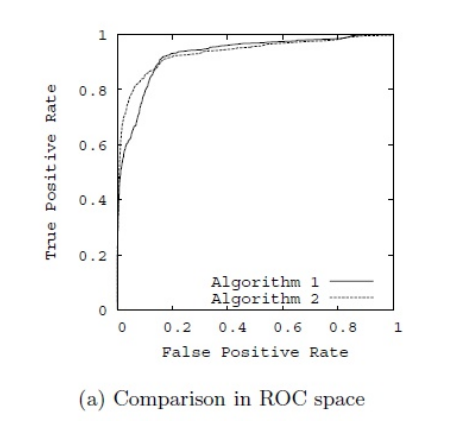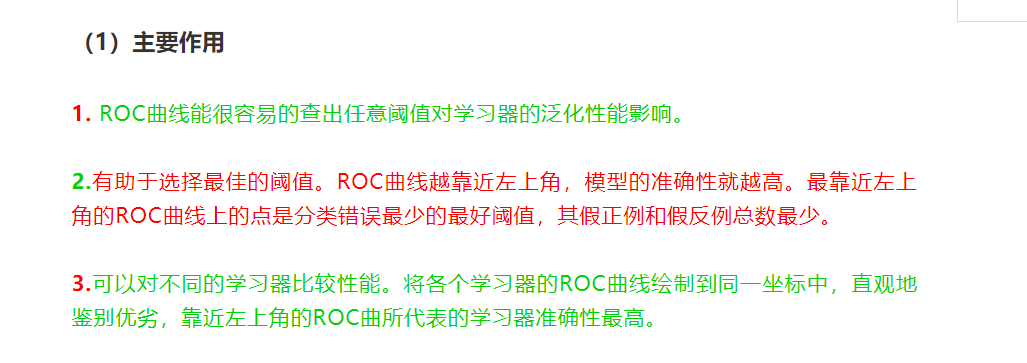PR curve:
A PR curve corresponds to a threshold. By selecting an appropriate threshold, such as 50%, the samples are divided, the probability greater than 50% is considered a positive example, and the probability less than 50% is a negative example, so as to calculate the corresponding precision rate and recall rate.

### Guess you like

Origin blog.csdn.net/weixin_45942265/article/details/119419236
Recommended
Ranking
Daily